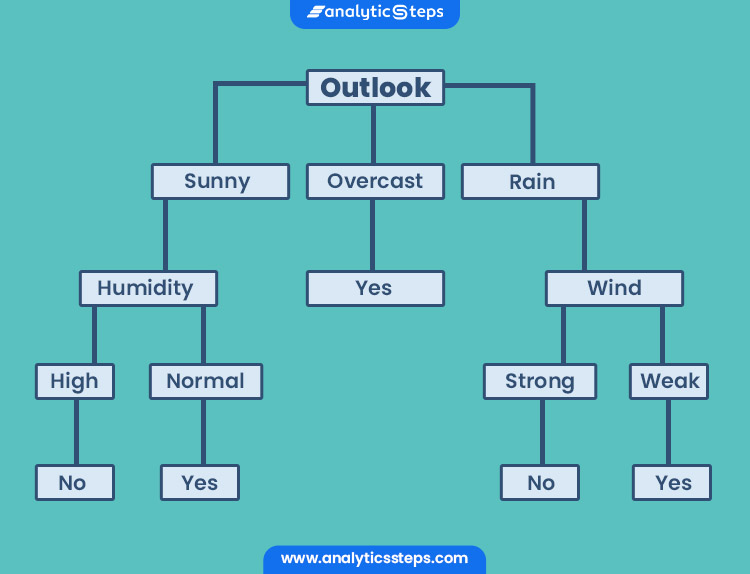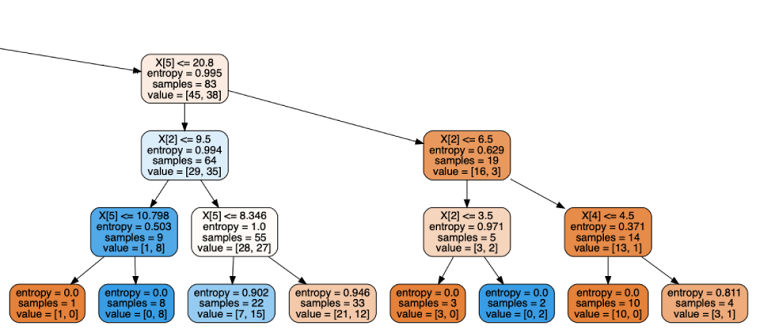# Introduction to Decision Tree Algorithm in Machine Learning

• Rohit Dwivedi
• May 10, 2020
• Machine Learning
• Updated on: Feb 10, 2021“The possible solutions to a given problem emerge as the leaves of a tree, each node representing a point of deliberation and decision.” -  Niklaus Wirth (1934 — ), Programming language designer

Decision trees are considered to be widely used in data science. It is a key proven tool for making decisions in complex scenarios. In Machine learning, ensemble methods like decision tree, random forest are widely used. Decision trees are a type of supervised learning algorithm where data will continuously be divided into different categories according to certain parameters.

So in this blog, I will explain the Decision tree algorithm. How is it used? How it functions will be covering everything that is related to the decision tree.

## What is a Decision Tree?

Decision tree as the name suggests it is a flow like a tree structure that works on the principle of conditions. It is efficient and has strong algorithms used for predictive analysis. It has mainly attributes that include internal nodes, branches and a terminal node.

Every internal node holds a “test” on an attribute, branches hold the conclusion of the test and every leaf node means the class label. This is the most used algorithm when it comes to supervised learning techniques.

It is used for both classifications as well as regression. It is often termed as “CART” that means Classification and Regression Tree. Tree algorithms are always preferred due to stability and reliability.

### How can an algorithm be used to represent a tree

Let us see an example of a basic decision tree where it is to be decided in what conditions to play cricket and in what conditions not to play.Decision Tree for playing cricket

You might have got a fair idea about the conditions on which decision trees work with the above example. Let us now see the common terms used in Decision Tree that is stated below:

• Branches - Division of the whole tree is called branches.

• Root Node - Represent the whole sample that is further divided.

• Splitting - Division of nodes is called splitting.

• Terminal Node - Node that does not split further is called a terminal node.

• Decision Node -  It is a node that also gets further divided into different sub-nodes being a sub node.

• Pruning - Removal of subnodes from a decision node.

• Parent and Child Node - When a node gets divided further then that node is termed as parent node whereas the divided nodes or the sub-nodes are termed as a child node of the parent node.

## How Does Decision Tree Algorithm Work

It works on both the type of input & output that is categorical and continuous. In classification problems, the decision tree asks questions, and based on their answers (yes/no) it splits data into further sub branches.

It can also be used as a binary classification problem like to predict whether a bank customer will churn or not, whether an individual who has requested a loan from the bank will default or not and can even work for multiclass classifications problems. But how does it do these tasks?

In a decision tree, the algorithm starts with a root node of a tree then compares the value of different attributes and follows the next branch until it reaches the end leaf node. It uses different algorithms to check about the split and variable that allow the best homogeneous sets of population.

"Decision trees create a tree-like structure by computing the relationship between independent features and a target. This is done by making use of functions that are based on comparison operators on the independent features."

## Types of Decision Tree

Type of decision tree depends upon the type of input we have that is categorical or numerical :

1. If the input is a categorical variable like whether the loan contender will defaulter or not, that is either yes/no. This type of decision tree is called a Categorical variable decision tree, also called classification trees.

2. If the input is numeric types and or is continuous in nature like when we have to predict a house price. Then the used decision tree is called a Continuous variable decision tree, also called Regression trees.Decision Tree Machine Learning Algorithm

### Lists of Algorithms

• ID3 (Iterative Dicotomizer3) – This DT algorithm was developed by Ross Quinlan that uses greedy algorithms to generate multiple branch trees. Trees extend to maximum size before pruning.

• C4.5 flourished ID3 by overcoming restrictions of features that are required to be categorical. It effectively defines distinct attributes for numerical features. Using if-then condition it converts the trained trees.

• C5.0 uses less space and creates smaller rulesets than C4.5.

• The CART classification and regression tree are similar to C4.5 but it braces numerical target variables and does not calculate the rule sets. It generates a binary tree.

(Recommend blog: Regression Techniques )

## How to prevent overfitting through regularization?

There is no belief that is assumed by the decision tree that is an association between the independent and dependent variables. Decision tree is a distribution-free algorithm. If decision trees are left unrestricted they can generate tree structures that are adapted to the training data which will result in overfitting.

To avoid these things, we need to restrict it during the generation of trees that are called Regularization. The parameters of regularization are dependent on the DT algorithm used.

### Some of the regularization parameters

1. Max_depth: It is the maximal length of a path that is from root to leaf. Leaf nodes are not split further because they can create a tree with leaf nodes that takes many inspections on one side of the tree whereas nodes that contain very less inspection get again split.

2. Min_sample_spilt: It is the limit that is imposed to stop the further splitting of nodes.

3. Min_sample_leaf: A min number of samples that a leaf node has. If leaf nodes have only a few findings it can then result in overfitting.

4. Max_leaf_node: It is defined as the max no of leaf nodes in a tree. (Relatable article: What are the Model Parameters and Evaluation Metrics used in Machine Learning?)

5. Max_feature_size:  It is computed as the max no of features that are examined for the splitting for each node.

6. Min_weight_fraction_leaf: It is similar to min_sample_leaf that is calculated in the fraction of total no weighted instances.

You can refer here to check about the usage of different parameters used in decision tree classifiers.

• Decision tree algorithm is effective and is very simple.

• Decision tree algorithms can be used while dealing with the missing values in the dataset.

• Decision tree algorithms can take care of numeric as well as categorical features.

• Results that are generated from the Decision tree algorithm does not require any statistical or mathematics knowledge to be explained.

• Logics get transformed if there are even small changes in training data.

• Larger trees get difficult to interpret.

• Biased towards three having more levels.

To see the documentation of the decision tree using the sklearn library, you can refer here.

## Conclusion

In Machine learning and Data science, you cannot always rely on linear models because there is non-linearity at maximum places. It is noted that tree models like Random forest, Decision trees deal in a good way with non-linearity.

Decision tree algorithms come from supervised learning models that can be used for both classification and regression tasks. The task that is challenging in decision trees is to check about the factors that decide the root node and each level, although the results in DT are very easy to interpret.

In this blog, I have covered what is the decision tree, what is the principle behind DT, different types of decision trees, different algorithms that are used in DT, prevention of overfitting of the model hyperparameters and regularization.

0%

•#### 360digitmgdatascience

Sep 08, 2020

I feel very grateful that I read this. It is very helpful and very informative and I really learned a lot from it. <a rel="nofollow" href="https://360digitmg.com/india/pmp-certification-course-training-in-visakhapatnam">360DigiTMG</a>

•